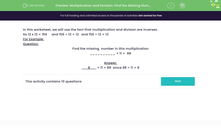# Multiplication and Division: Find the Missing Number

In this worksheet, students continue to become familiar with the fact that division is the inverse of multiplication.Key stage:  KS 2

Curriculum topic:   Number: Multiplication and Division

Curriculum subtopic:   Solve Multiplication/Division Problems

Difficulty level:#### Worksheet Overview

In this worksheet, we will use the fact that multiplication and division are inverses.

So 12 x 13 = 156     and 156 ÷ 13 = 12   and 156 ÷ 12 = 13

For Example:

Question:

Find the missing  number in this multiplication

__________ × 11 =  88